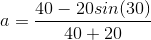# SAT II Physics : Kinematics Equations

## Example Questions

### Example Question #14 : Using Motion Equations

An egg falls from a nest in a tree that is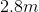tall. A girl,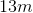away, runs to catch the egg. If she catches it right at the moment before it hits the ground, how fast does she need to run?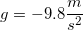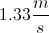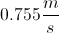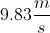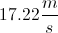Explanation:

The important thing to recognize here is that the amount of time the egg is falling will be equal to the amount of time the girl is running.

Our first step will be to find the time that the egg is in the air.

We know it starts from restabove the ground, and we know the gravitational acceleration. Its total displacement will be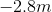, since it falls in the downward direction. We can use the appropriate motion equation to solve for the time: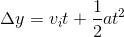Use the given values in the formula to solve for the time.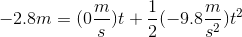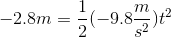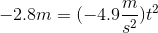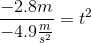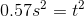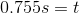Now that we have the time, we can use it to find the speed of the girl. Her speed will be determined by the distance she travels in this amount of time.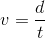Use our values for her distance and the time to solve for her velocity.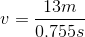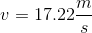### Example Question #1 : Mechanics

A ball is dropped from the roof a building that is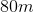tall. How long will it be before the ball hits the ground?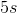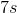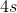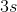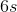Explanation:

We can solve this problem using the kinematics equations. Note that the initial velocity will be zero, since the ball is dropped, and the acceleration will be equal to the acceleration of gravity.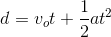This can be simplified, since the initial velocity is zero.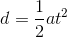Use the given value for the distance (the height of the building) and the acceleration of gravity to solve for the time.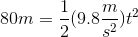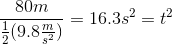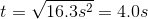### Example Question #1 : Kinematics Equations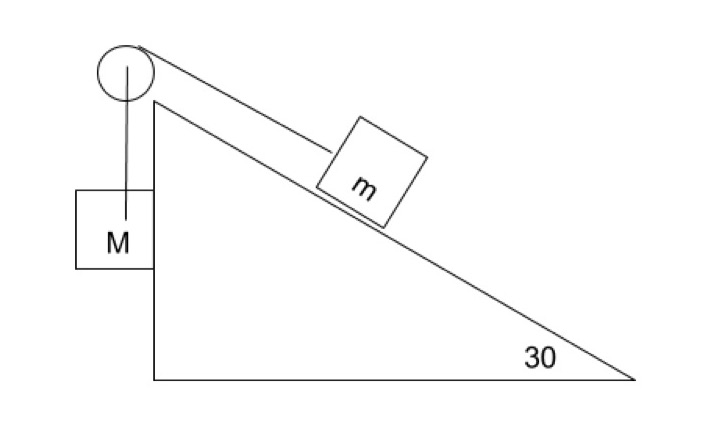If the block of massslides up a frictionless incline plane and is pulled by masswhich is falling then what is the acceleration of the block on the ramp if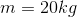and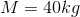and the angle of incline is 30 degrees?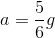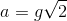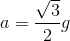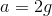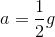Explanation:

Begin by making a free body diagram for each block: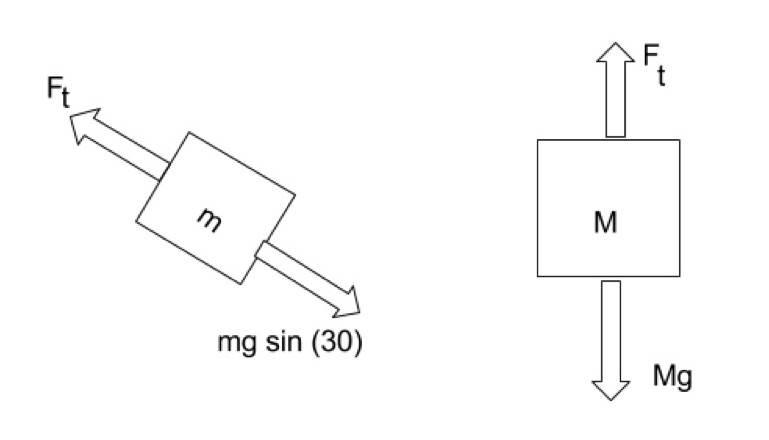Use the diagram to write an equation for net force on each block: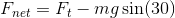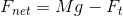Since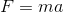for the block on the ramp and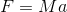for falling block we can substitute into these equations: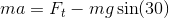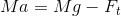Then add the equations to get: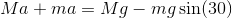Next rearrange to isolate: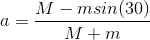Substitute the given values from the question and solve: